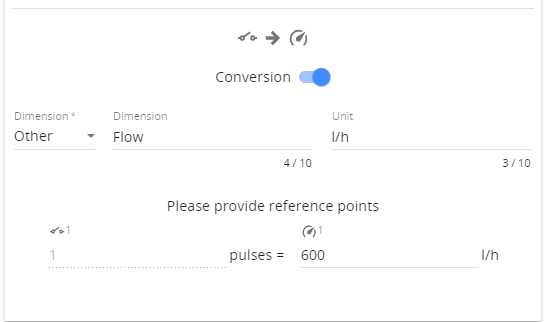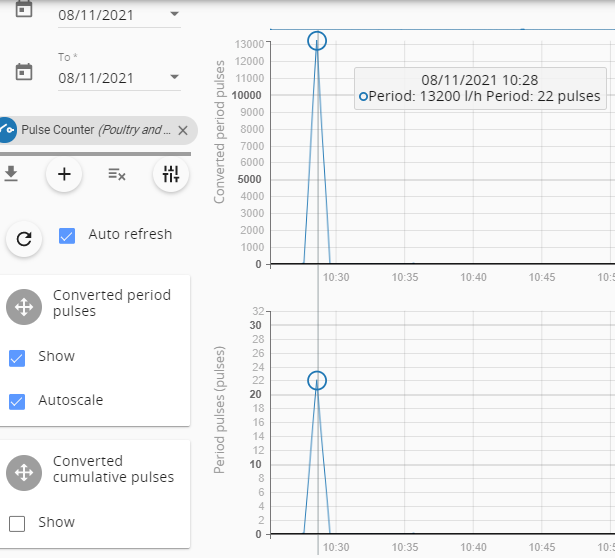How to configure Aranet Pulse Counter sensor for flow measurement

•Aranet Pulse Counter sensor https://aranet.com/product/aranet-pulse-counter-sensor/ counts the times that a mechanical contact closes. This allows to use it with liquid volume counter having pulse output for flow measurement.

Let's take for example water meter with a reed switch providing 1 pulse on every 10 liters of water.
When paired to Aranet Pro base Pulse counter sensor should be configured with conversion:
one pulse is configured as (10 liters x 60 seconds) / measurement interval. So for a one-minute measurement interval, one impulse will correspond to 600 liters/hour, 2 minutes: 300 liter/hour, 5 minutes: 120 liters/hour, and 10 minutes: 60 liters/hour. Then converted period pulses measurement can be checked to see the correct water flow rate per each measurement interval:During the measurement interval, the sensor counts pulses and at the end of the interval sends data to Aranet Pro base station. Then resets the pulse counter and starts counting again for the new measurement interval.

For example, if 22 pulses were counted in the 1-minute interval and each pulse corresponds to 10 l water volume then the flow rate for one hour will be 22 x 10 x 60 = 13 200 l/h:You should note that the smaller is the measurement interval, the lower is the accuracy: one pulse in shorter intervals has a bigger value than in longer intervals. Thus one pulse introduced uncertainty/inaccuracy is also higher. One minute measurement interval for 10 l water volume per pulse should correspond to 600 l/h, but for 10-minute measurement interval, it will be just 60 l/h. So with a higher measurement interval, more pulses could be counted and a more precise flow rate value be obtained on the measurements.